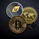# Binary option trading by two previous bars

3741 views
3741
This simple script uses the idea of inertia of the market. if 2 previous candles have the same color, current meant to have that too. Following this signal is equal to buying a binary option on the start of the bar (week here). Signals are shown as arrows on the series. The color of the bar shows the outcome of the current option: yellow is success, black is failure. The same outcomes are at the bottom of the chart. The blue line is the total revenue of all options so far. Can be used as template for strategy simulation.
```study(title="trade by two-bars", shorttitle="by2bars", overlay=true)

data = (close>open) == (close>open)
dir = close < close
correct = (close < close) == (open<close)

plotshape(data and dir, color=lime, style=shape.arrowup, text="Buy", location=location.abovebar )
plotshape(data and not dir, color=red, style=shape.arrowdown, text="Sell", location=location.belowbar)

barcolor(data and correct?yellow:(data?black:na))

yellow_bars = (data and correct)
black_bars = -(data and not correct)

plot(yellow_bars,"success",green,8,histogram)
plot(black_bars,"failure",red,8,histogram)

yellow_bars_so_far = cum(yellow_bars)
black_bars_so_far = cum(black_bars)
total_revenue = yellow_bars_so_far + black_bars_so_far
plot(total_revenue,"total revenue",blue)```

## Kommentarecandles are cramped up any idea how to fix that ?
Antworten